Solubility of Salts & Acid Base Indicators

# Solubility of Salts & Acid Base Indicators - Notes | Study Physical Chemistry - Chemistry

 1 Crore+ students have signed up on EduRev. Have you?

28. Preferential Precipitation of Salts.

Frequently, a solution conations more than one ion capable of forming a ppt. with another ion which is added to the solution. e.g., in a solution containing Cl, Br– and I, if Ag+ ions are added then out of the three, the least soluble silver salt is ppt first. If the addition of Ag+ ions is continued, eventually a stage is reached when the next lesser soluble salt starts ppt along with the least soluble salt and so on if the stoichiometry of the ppted salts is the same, then the salt with the minimum Ksp or minimum solubility will ppted first followed by higher Ksp

If the stoichiometry of the ppted salts is not the same, then with Ksp alone, we can’t predict which ion will ppted first e.g. a solution containing Cl– and CrO42– both of these ions from ppt with Ag+ through the Ksp(AgCl ) > Ksp (Ag2CrO4). Yet it is AgCl (less soluble) which ppted first when Ag+ ions added to the solution. In order to predict which ion (Cl or CrO42–) ppt first. We have to calculate the conc. of Ag+ ion needed to start ppt through the Ksp and given conc. of Cl and CrO42– if the conc. of Ag+ ions needed to start the ppt of CrO42– is large than that of Cl. Hence as AgNOis added to the solution, the minimum of the two conc. of Ag+ to start the ppt will be reached first and thus the corresponding ion (Cl in this case) will be ppted in preference to the other. During the course of ppt conc. of Cl decreases and conc. of Ag+ increases when it’s conc. become equals to the conc. required (of Ag+) for CrO42–. At this stages the whole of Cl ions have been ppted the addition of more of AgNO3 causes the ppt of both the ions together.

(i) Solubility of a salt of weak acid and strong base in Basic Buffer suppresses than pure water due to common ion effect. But in acidic buffer solution solubility increase than pure water.

(ii) Solubility of salt of weak acid and weak base in pure water: Let the solubility of salt be S, and y mol/litre is the amount of salt getting hydrolyzed.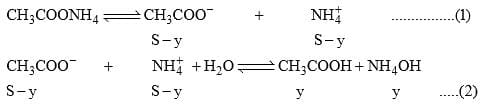Ksp = (S – y) (S – y) = (S – y)

Due to hydrolysis of salt from equation (2)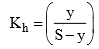And we also know that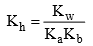29. Solubility of a salt of weak acid and weak base in acidic buffer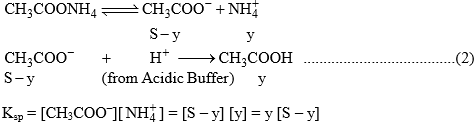For equation (2)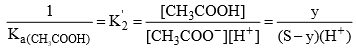Solubility of CH3COONH4 in acidic buffer would be higher than in pure water.

30. Solubility of a salt of weak acid and weak base in basic buffer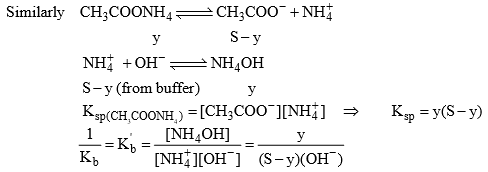The solubility of CH3COONH4+ in basic buffer would be higher than pure water.

31. Solubility of AgCl in an aqueous solution containing NH3

Let the amount of NH3 initially be ‘a’ M. If the solubility of salt be ‘b’ mole/litre.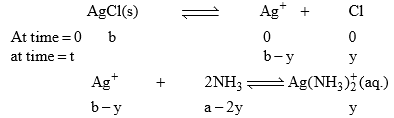Where y is the amount of Ag+ which reacted with NH3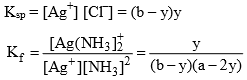After knowing the value of Ksp and Kf the value of solubility can be calculated.

32. Acid-base Indicators

An acid & indicator are substance which changes it’s color within limits with variation in pH of the solution to which it is added. Indicators, in general are either organic weak acid or weak bases with a characteristics of having different colours in the ionized and unionized form e.g. phenolphthalein is a weak acid (ionized from in pink and unionized form is colourless).

Acidic Indicator Action (e.g. HPh)

HPh (Phenolphthalein) is a colouless weak acid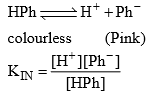If the solution is acidic, the H+ by the acid increases and since Kin is constant and it does not depend upon the concentration so HPb increases mean equilibrium will shift towards left means solution remain colourless. By addition of alkali, OH– will be furnished and that OH will combines will H+ of HPh to form water and equilibrium will moves towards right and therefore solution becomes pink. Thus HPh appears colourless in acidic and pink in alkaline solution pH range of HPh is (8.3 – 10).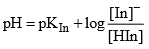The colour of the indicator changes from colour A to colour B at a particular point known as end point of indicator. At this point [HIn] = [In] means pH = pKIn (at this point half of indicator is in the acid form and half in the form of its conjugate form.

33. Indicator (Basic) action of MeOH (Methyl Orange)

When MeOH is dissolved in water and undergoes dissociation to a small extent. The undissociated molecules are yellow while dissociated Me+ are red in colour.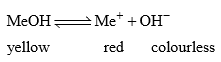If the solution is acidic, the H+ furnished by the acid combines with OH ions furnished by the indicators to form undissociated water. This shifts the equilibrium towards right giving red coloured solution. Therefore in acid solution, this indicator gives red colour. In the presence of alkali, OH increases and due to common ion effect the dissociation of MeOH surpress means equilibrium will shifts towards left. Hence the solution in alkaline medium remains yellow in colour.

Colour of solution depends upon relative amount of ionized form to unionized form (ratio of Me MeOH).

In general pH range of indicator lies B/w pKln –1 to pKln + 1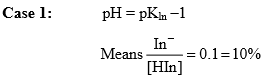Percentage ionization of indicator would be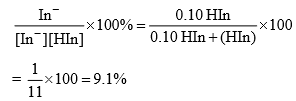In fact, pH = pKln –1 is the maximum pH upto which the solution has a distinct colour characteristic of HIn. At pH smaller than this value, one of the indicator is present in the unionized form.  Thus at pH ≤ pKln –1, the solution has a colour characteristic of HIn.

Case 2: At pH = pKln +1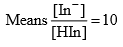Percentage of ionization of indicator is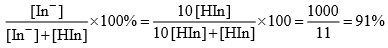Thus most of the indicator is present in the ionized form In and solution gets the colour characteristic. Infect pH = pKln + 1 is the minimum pH upto which the solution has a distinct characteristic of In. At pH greater than this value, still more of the indicator is present in the ionized form. Thus at pH ≥ pKln +1, the solution has a colour characteristic of In.

34. Ostwald’s Theory:

According to this theory:

(a) The colour change is due to ionization of the acid-base indicator. The unionized form has different colour than the ionized form.

(b) The ionization of the indicator is largely affected in acids and bases as it is either a weak acid or a weak base. In case, the indicator is a weak acid, its ionization is very much low in acids due to common H+ ions while it is fairly ionized in alkalies. Similarly if the indicator is a weak base, its ionization is large in acids and low in alkalies due to common OH ions.

Considering two important indicators phenolphthalein (a weak acid) and methyl orange (a weak base), Ostwald theory can be illustrated as follows:

Phenolphthalein: It can be represented as HPh. It ionizes in solution to a small extent as: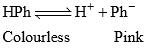Applying law of mass action,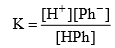The undissociated molecules of phenolphthalein are colourless while Ph ions are pink in colour. In presence of an acid, the ionization of HPh is practically negligible as the equilibrium shifts to left hand side due to high concentration of H+ ions. Thus, the solution would remain colourless. On addition of alkali, hydrogen ions are removed by OH ions in the form of water molecules and the equilibrium  shifts to right hand side. Thus, the concentration of Ph ions increases in solution and they impart pink colour to the solution.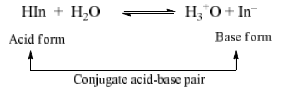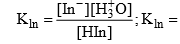Ionization constant of indicator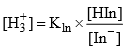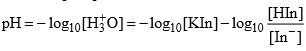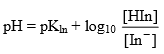(Handerson equation for indicator)

[In] = [HIn] and pH = pKln

Methyl Orange:  It is a weak base and can be represented as MeOH. It is ionized in solution to give Me and OH ions.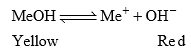Applying law of mass action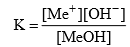In presence of an acid, OH, ions are removed in the form of water molecule and the above equilibrium shifts to right hand side. Thus, sufficient Me+ ions are produced which impart red colour to the solution. On addition of alkali, the concentration of OH ions increases in the solution and the equilibrium shifts to left hand side (due to common ion effect), i.e., the ionization of MeOH is practically negligible. Thus, the solution acquires the colour of unionized methyl orange molecules, i.e., yellow.

This theory also explains the reason why phenolphthalein is not a suitable indicator for titrating a weak base against strong acid. The OH ions furnished by a weak base are not sufficient to shift the equilibrium towards right hand side considerably, i.e., pH is not reached to 8.3. Thus, the solution does not attain pink colour. Similarly, it can be explained why methyl orange is not a suitable indicator for the titration of weak acid with strong base.

Quinonoid Theory:  According to this theory:

(a) The acid base indicators exist in two tautomeric forms having different structures. Two forms are in equilibrium. One form is termed benzenoid form and the other quinonoid form.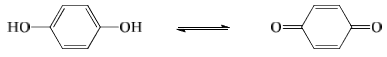(b) The two forms have different colours. The colour change is due to the interconversion of one tautomeric form into other.

(c) One form mainly exists in acidic medium and the other in alkaline medium.

Thus, during titration the medium changes from acidic to alkaline or vice-versa. The change in pH converts one tautomeric form into other thus, the colour change occurs.

Phenolphthalein has benzenoid form in acidic medium and thus, it is colourless while it has quinonoid form in alkaline medium which has pink colour.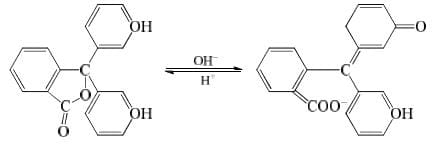Methyl orange has quinonoid form in acidic solution and benzenoid form in alkaline solution. The colour of benzenoid form is yellow while that of quinonoid form is red.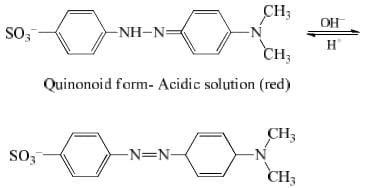The document Solubility of Salts & Acid Base Indicators - Notes | Study Physical Chemistry - Chemistry is a part of the Chemistry Course Physical Chemistry.
All you need of Chemistry at this link: Chemistry

## Physical Chemistry

85 videos|131 docs|67 tests
 Use Code STAYHOME200 and get INR 200 additional OFF

## Physical Chemistry

85 videos|131 docs|67 tests

Track your progress, build streaks, highlight & save important lessons and more!

,

,

,

,

,

,

,

,

,

,

,

,

,

,

,

,

,

,

,

,

,

;